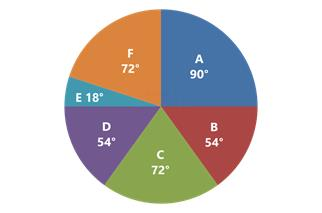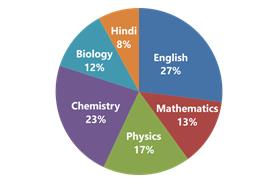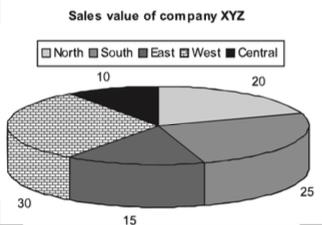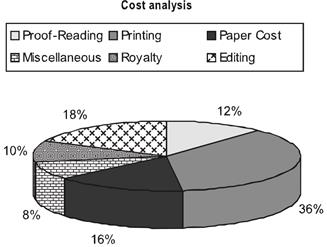Courses

# Pie Charts CAT Notes | EduRev

## CAT : Pie Charts CAT Notes | EduRev

The document Pie Charts CAT Notes | EduRev is a part of CAT category.
All you need of CAT at this link: CAT

Learning Objectives

• You will get an understanding of what are Pie Charts and a first-hand view of how data is presented through its organisation in the form of Pie charts.
• You will get an understanding of typical data which can be represented through Pie charts. Also, understand the concept of how the distribution of a whole is done in the form of Pie Charts.
• You will get a first hand feel of the typical question types that are asked on the basis of Pie charts.
• Practice exercise on Pie charts containing simulated examination questions

Introduction

Pie charts are specific types of data presentation where the data is represented in the form of a circle. In a pie chart, a circle is divided into various sections or segments such that each sector or segment represents a certain proportion or percentage of the total. In such a diagram, the total of all the given items is equated to 360 degrees and the degrees of angles, representing different items, are calculated proportionately. The entire diagram looks like a pie and its components resemble slices cut from a pie. The pie chart is used to show the break-up of one continuous variable into its component parts.

The types of pie charts

There are two approaches to constructing a pie chart from any given data
(A) Degree Approach: The central angle in a circle represents 360o, so any part or segment in a pie chart is calculated as a proportion of 360o.(B) Percentage Approach: In this case, any part or segment in a pie chart is calculated as a part of 100%.Conversion of degree approach to percentage approach:If we convert the same pie chart into the degree format, we will be required to do the following conversions:
Total = 100%
Hence, 1% = 3.6o
Central = 10% = 36o
North = 20% = 72o
South = 25% = 90o
East = 15% = 54o
West =30% = 108o

Try yourself:The central angle of a sector is 72o. What percentage of the circle is comprised by the sector?

Significance of Pie Charts

The pie chart has gained prominence due to the following reasons:

• In a pie chart, we get a clear picture of the contribution of different sectors to the build up of the total. E.g., presentation of budgets.
• Comparing two pie charts is easier than comparing two bar charts or any other format of data representation.

Example: Following is the cost analysis of a book “Guide to Digital Marketing”.What is the central angle showing the cost of paper?

(a) 42.8o
(b) 32.6o
(c) 36.8o
(d) 57.6o
Solution: Percentage of paper cost in total cost - 16%
We know that the sum of angles in pie chart is 100o
Hence, Required Angle = (16/100 × 360) = 57.6o

Try yourself:If 5500 copies of the book are published and the miscellaneous expenditure amounts to Rs.36,960 and the marked price is 40% above the cost price, then the marked price of each copy is

Offer running on EduRev: Apply code STAYHOME200 to get INR 200 off on our premium plan EduRev Infinity!

,

,

,

,

,

,

,

,

,

,

,

,

,

,

,

,

,

,

,

,

,

;